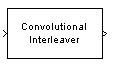# Convolutional Interleaver

Permute input symbols using set of shift registers

## Library

Convolutional sublibrary of Interleaving

•## Description

The Convolutional Interleaver block permutes the symbols in the input signal. Internally, it uses a set of shift registers. For information about delays, see Delays of Convolutional Interleaving and Deinterleaving.

The Initial conditions parameter indicates the values that fill each shift register at the beginning of the simulation (except for the first shift register, which has zero delay). If Initial conditions is a scalar, then its value fills all shift registers except the first; if Initial conditions is a column vector whose length is the Rows of shift registers parameter, then each entry fills the corresponding shift register. The value of the first element of the Initial conditions parameter is unimportant, since the first shift register has zero delay.

This block accepts a scalar or column vector input signal, which can be real or complex. The output signal has the same sample time as the input signal.

The block can accept the data types `int8`, `uint8`, `int16`, `uint16`, `int32`, `uint32`, `boolean`, `single`, `double`, and fixed-point. The data type of this output will be the same as that of the input signal.

## Parameters

Rows of shift registers

The number of shift registers that the block uses internally.

Register length step

The number of additional symbols that fit in each successive shift register, where the first register holds zero symbols.

Initial conditions

The values that fill each shift register when the simulation begins.

## Examples

For an example that uses this block, see Convolutional Interleaving.

## Pair Block

Convolutional Deinterleaver